# RF Noise Modeling

This example shows how to use the RF Blockset™ Circuit Envelope library to simulate noise and calculate noise power. Results are compared against theoretical calculations and a Communications Toolbox™ reference model.

### System Architecture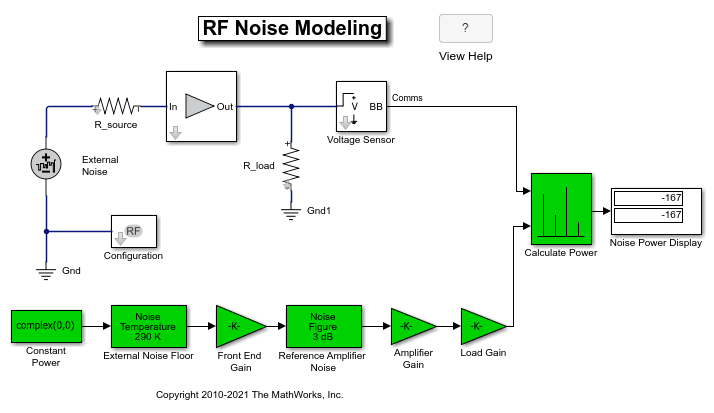The model defines variables for block parameters using the PreloadFcn callback function. To access model callbacks, select MODELING > Model Settings > Model Properties and select the Callbacks tab in the Model Properties window.

The RF system, shown in white, consists of these blocks.

• Configuration block - This block sets global simulation parameters for the RF Blockset system. Selecting Simulate Noise adds noise to the simulation.

• External Noise block - This block adds a power spectral density of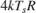at the input. In this equation,is the Boltzmann constant,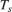is the temperature of the source, andis the noise reference impedance. The calculated noise level of -174 dBm/Hz is used in this example. The External Noise block provides an explicit signal source.

• Amplifier block - This block specifies the power gain and noise figure.

• Voltage Sensor block - This is an Outport block with the Source type parameter set to Ideal voltage.

• Resistor blocks - These blocks specify the source and load resistance.

The Communications Toolbox reference system, shown in green, consists of these blocks.

• Constant Power block - This block provides a constant input signal source.

• Gain blocks - These blocks model front end gain, amplifier gain and loading effects.

• Receiver Thermal Noise blocks - These blocks model the external noise floor and the reference amplifier noise, respectively.

The Calculate Power block computes RMS noise power for the actual load resistance, R_load.

### Run Example

1. Use the Open Model button to open and run the model.

The Noise Power Display block verifies that the RF Blockset and Communications Toolbox noise models are equivalent.

### Compute RF System Noise

To enable noise in the RF Blockset circuit envelope environment:

• In the Configuration block dialog, select Simulate noise.

• Specify a Temperature. RF Blockset uses this value to calculate the equivalent noise temperature inside the amplifier.

• Specify the Noise figure (dB) parameter of any amplifiers or mixers in the system.

In the example, for a specified LNA gain of 4 dB and noise figure of 3 dB, the output noise is calculated using the following equations: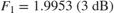The next equation converts the noise factor to an equivalent noise temperature.is the Temperature parameter of the RF Blockset Configuration block.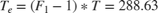The final equation calculates the output noise power.is the temperature of the SimRF™ External Noise block and the Communications Toolbox External Noise Floor block.The available noise power is the power that can be supplied by a resistive source when it is feeding a noiseless resistive load equal to the source resistance. The green External Noise Floor block generates an available power referenced to 50 ohms.

The Front End Gain block models the voltage divider due to the source resistance and the input impedance of the amplifier.

The green Reference Amplifier Noise and Amplifier Gain blocks model the noise added by the amplifier and the amplifier gain, respectively.

The output of the green Amplifier Gain block is equal to the voltage across the RF Blockset R_load block.